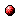# Model predictive control for stochastic max-min-plus-scaling systems - An approximation approach

Reference:
S.S. Farahani, T. van den Boom, and B. De Schutter, "Model predictive control for stochastic max-min-plus-scaling systems - An approximation approach," Proceedings of the 2011 50th IEEE Conference on Decision and Control and European Control Conference (CDC-ECC), Orlando, Florida, pp. 391-396, Dec. 2011.

Abstract:
A large class of discrete-event and hybrid systems can be described by a max-min-plus-scaling (MMPS) model, i.e., a model in which the main operations are maximization, minimization, addition, and scalar multiplication. Further, Model Predictive Control (MPC), which is one of the most widely used advanced control design methods in the process industry due to its ability to handle constraints on both inputs and outputs, has already been extended to both deterministic and stochastic MMPS systems. However, in order to compute an MPC controller for a general MMPS system, a nonlinear, nonconvex optimization problem has to be solved. In addition, for stochastic MMPS systems, the problem is computationally highly complex since the cost function is defined as the expected value of an MMPS function and its evaluation leads to a complex numerical integration. The aim of this paper is to decrease this computational complexity by applying an approximation method that is based on the raw moments of a random variable, to a stochastic MMPS system with a Gaussian noise. In this way, the problem can be transformed into a sequence of convex optimization problems, providing that linear or convex MPC input constraints are considered.Corresponding technical report: pdf file (153 KB)
Note: More information on the pdf file format mentioned above can be found here.

Bibtex entry:

@inproceedings{Farvan:11-040,
author={S.S. Farahani and T. van den Boom and B. {D}e Schutter},
title={Model predictive control for stochastic max-min-plus-scaling systems -- {An} approximation approach},
booktitle={Proceedings of the 2011 50th IEEE Conference on Decision and Control and European Control Conference (CDC-ECC)},